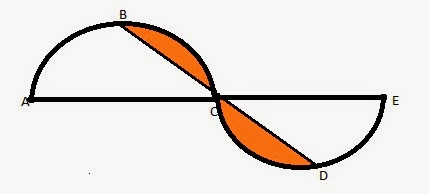# GMAT Practice Questions - Geometry

Must solve GMAT practice questions in Geometry. The following topics are covered in the GMAT quant section from Geometry:

1. Triangles
2. Lines and angles
1. Circles
2. Coordinate Geometry
3. Solids

Click on the Problem Solving or the Data Sufficiency tab for practice questions. The topic from which the question has been selected and the concept tested are listed in the right column for each question. Detailed explanatory answers, solution videos and slide decks are also provided.

• 1
• In the figure given below, ABC and CDE are two identical semi-circles of radius 2 units. B and D are the mid points of the arc ABC and CDE respectively. What is the area of the shaded region?A. 4π - 1
B. 3π - 1
C. 2π - 4
D. ½(3π - 1)
E. 2π - 2

Solution

• 1
• a, b, and c are sides of a right triangle. What is the area of the triangle? Statement 1: a = 4.
Statement 2: a + b + c = 4.
• Geometry
• Right Triangles